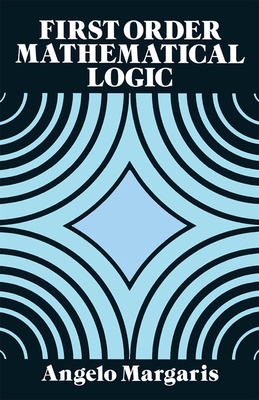Discounted# First Order Mathematical Logic (Dover Books on Mathematics)

Previous price: \$15.00 Current price: \$12.95
This product is not returnable.
Publication Date: March 1st, 1990
Publisher:
Dover Publications
ISBN:
9780486662695
Pages:
224
Special Order - Subject to Availability

## Description

"Attractive and well-written introduction." -- Journal of Symbolic Logic
The logic that mathematicians use to prove their theorems is itself a part of mathematics, in the same way that algebra, analysis, and geometry are parts of mathematics. This attractive and well-written introduction to mathematical logic is aimed primarily at undergraduates with some background in college-level mathematics; however, little or no acquaintance with abstract mathematics is needed.
Divided into three chapters, the book begins with a brief encounter of na ve set theory and logic for the beginner, and proceeds to set forth in elementary and intuitive form the themes developed formally and in detail later. In Chapter Two, the predicate calculus is developed as a formal axiomatic theory. The statement calculus, presented as a part of the predicate calculus, is treated in detail from the axiom schemes through the deduction theorem to the completeness theorem. Then the full predicate calculus is taken up again, and a smooth-running technique for proving theorem schemes is developed and exploited.
Chapter Three is devoted to first-order theories, i.e., mathematical theories for which the predicate calculus serves as a base. Axioms and short developments are given for number theory and a few algebraic theories. Then the metamathematical notions of consistency, completeness, independence, categoricity, and decidability are discussed, and the predicate calculus is proved to be complete. The book concludes with an outline of Godel's incompleteness theorem.
Ideal for a one-semester course, this concise text offers more detail and mathematically relevant examples than those available in elementary books on logic. Carefully chosen exercises, with selected answers, help students test their grasp of the material. For any student of mathematics, logic, or the interrelationship of the two, this book represents a thought-provoking introduction to the logical underpinnings of mathematical theory.
"An excellent text." -- Mathematical Reviews